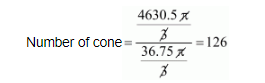# A metallic sphere of radius 10.5 cm`
Question:

A metallic sphere of radius 10.5 cm is melted and then recast into small cones, each of radius 3.5 cm and height 3 cm. The number of such cones is

(a) 63

(b) 126

(c) 21

(d) 130

Solution:

Radius of metallic sphere = 10.5 cm

Therefore,

Volume of the sphere

$=\frac{4}{3} \pi r^{3}$'

$=\frac{4}{3} \times \pi \times 10.5 \times 10.5 \times 10.5$

$=\frac{4630.5 \pi}{3}$............(1)

Now,

Radius of the cone = 3.5 cm

and Height of the cone = 3 cm

Therefore,

Volume of the cone

$=\frac{1}{3} \pi r^{2} h$

$=\frac{1}{3} \times \pi \times 3.5 \times 3.5 \times 3$

$=\frac{36.75 \pi}{3}$.......... (ii)

Number of cone $=\frac{\text { volume of sphere }}{\text { volume of cone }}$

Dividing eq. (i) and (ii) we getNumber of cone = 126

Hence, the correct answer is choice (b).